# Data Preprocessing in R Studio: Exercises and Solutions

In order to solve the tasks you need:

The dataset for these exercises is called SalesData. The dataset is used to record the sales information of a company. The task is to preprocess sales data that will help the company uncover some characteristics of the sale.

## 1. Data Examination and Imputation

S0 <- read.csv("SalesData.csv", stringsAsFactors = TRUE)
1. Check the names and data types of variables (columns) in R.
str(S0)
## 'data.frame':    670 obs. of  12 variables:
##  $Order.Date : Factor w/ 395 levels "1/1/2011","1/10/2011",..: 34 29 29 290 344 344 260 260 172 144 ... ##$ Order.Priority  : Factor w/ 6 levels "Critical","High",..: 3 2 2 2 6 6 2 2 2 3 ...
##  $Order.Quantity : int 6 49 27 30 19 21 12 22 21 44 ... ##$ Sales           : num  262 10123 245 4966 394 ...
##  $Ship.Mode : Factor w/ 3 levels "Delivery Truck",..: 3 1 3 3 3 3 3 3 2 3 ... ##$ Profit          : num  -213.2 457.8 46.7 1199 30.9 ...
##  $Unit.Price : num 38.94 208.16 8.69 195.99 21.78 ... ##$ Shipping.Cost   : num  35 68.02 2.99 3.99 5.94 ...
##  $Customer.Name : Factor w/ 85 levels "Aaron Bergman",..: 71 9 9 30 22 22 19 19 70 35 ... ##$ Customer.Segment: Factor w/ 4 levels "Consumer","Corporate",..: 4 1 1 2 1 1 2 2 2 3 ...
##  $Product.Category: Factor w/ 3 levels "Furniture","Office Supplies",..: 2 2 2 3 2 1 2 2 2 2 ... ##$ company         : Factor w/ 1 level "A": 1 1 1 1 1 1 1 1 1 1 ...
1. Is there any variable(s) will yield no usable statistical or graphical information? If yes, list them and explain why they are of no use.

Variable company will yield no usable information, because it has only one value: A.

1. Identify the variable(s) that have missing data.
summary(S0)
##       Order.Date        Order.Priority Order.Quantity       Sales
##  10/9/2009 :  6   Critical     :132    Min.   :-10.00   Min.   :    4.99
##  5/17/2011 :  6   High         :171    1st Qu.: 12.00   1st Qu.:  166.76
##  8/10/2011 :  6   Low          :131    Median : 25.00   Median :  497.14
##  8/4/2010  :  6   Loww         :  2    Mean   : 26.08   Mean   : 1915.47
##  11/23/2010:  5   Medium       :100    3rd Qu.: 39.00   3rd Qu.: 1773.86
##  2/6/2010  :  5   Not Specified:132    Max.   :101.00   Max.   :26133.39
##  (Other)   :636   NA's         :  2                     NA's   :1
##           Ship.Mode       Profit            Unit.Price      Shipping.Cost
##  Delivery Truck: 90   Min.   :-11053.60   Min.   :   1.26   Min.   :  0.49
##  Express Air   : 65   1st Qu.:   -82.33   1st Qu.:   7.25   1st Qu.:  3.14
##  Regular Air   :513   Median :     3.89   Median :  22.78   Median :  6.50
##  NA's          :  2   Mean   :   193.54   Mean   :  95.42   Mean   : 13.76
##                       3rd Qu.:   161.82   3rd Qu.:  99.99   3rd Qu.: 15.10
##                       Max.   : 10951.31   Max.   :3499.99   Max.   :147.12
##                       NA's   :2           NA's   :2
##          Customer.Name       Customer.Segment        Product.Category company
##  Carlos Soltero : 26   Consumer      : 79     Furniture      :145     A:670
##  Doug Bickford  : 22   Corporate     :336     Office Supplies:362
##  Grant Carroll  : 20   Home Office   :132     Technology     :163
##  Jack Lebron    : 19   Small Business:123
##  Sylvia Foulston: 19
##  (Other)        :563
##  NA's           :  1

Variables Order.Priority, Sales, Ship.Mode, Profit, Unit.Price and Customer.Name have missing data.

1. Perform data imputation by following methods:

2. Delete all rows with missing data and name the new dataset as S1.

S1 <- na.omit(S0)
1. Replace the missing values with the mean value of each variable.
S0$Sales[is.na(S0$Sales)] <- mean(S0$Sales, na.rm = TRUE) S0$Profit[is.na(S0$Profit)] <- mean(S0$Profit, na.rm = TRUE)
S0$Unit.Price[is.na(S0$Unit.Price)] <- mean(S0$Unit.Price, na.rm = TRUE) Missing values for variables Order.Priority, Ship.Mode and Customer.Name cannot be replaced by the mean value, because these variables are categorical. 1. Replace the missing values with random value between min and max of each variable. Since missing values in S0 have been replaced with the mean value of each variable in part (ii), we need to import the dataset again to restore the missing values. S0 <- read.csv("SalesData.csv", stringsAsFactors = TRUE) Replacing the missing values for numerical variables: S0$Sales[is.na(S0$Sales)] <- runif(n = sum(is.na(S0$Sales)),
min = min(S0$Sales, na.rm = TRUE), max = max(S0$Sales, na.rm = TRUE))
S0$Profit[is.na(S0$Profit)] <- runif(n = sum(is.na(S0$Profit)), min = min(S0$Profit, na.rm = TRUE),
max = max(S0$Profit, na.rm = TRUE)) S0$Unit.Price[is.na(S0$Unit.Price)] <- runif(n = sum(is.na(S0$Unit.Price)),
min = min(S0$Unit.Price, na.rm = TRUE), max = max(S0$Unit.Price, na.rm = TRUE))

Since categorical variables do not have min and max values, we can replace the missing values for categorical variables by random value from each variable.

S0$Order.Priority[is.na(S0$Order.Priority)] <- sample(levels(S0$Order.Priority), size = sum(is.na(S0$Order.Priority)),
replace = TRUE)
S0$Ship.Mode[is.na(S0$Ship.Mode)] <- sample(levels(S0$Ship.Mode), size = sum(is.na(S0$Ship.Mode)),
replace = TRUE)
S0$Customer.Name[is.na(S0$Customer.Name)] <- sample(levels(S0$Customer.Name), size = sum(is.na(S0$Customer.Name)),
replace = TRUE)

Use the data set S1 for the following tasks.

## 2. Data Exploration I

1. For all the numeric variables, find the mean, median, standard deviation, min and max using R function summary() or other functions. Put the results in a table in your report, with the variables in alphabetical order.
summary(S1)
##       Order.Date        Order.Priority Order.Quantity       Sales
##  10/9/2009 :  6   Critical     :131    Min.   :-10.00   Min.   :    4.99
##  5/17/2011 :  6   High         :169    1st Qu.: 12.00   1st Qu.:  166.45
##  8/10/2011 :  6   Low          :130    Median : 25.50   Median :  500.89
##  8/4/2010  :  6   Loww         :  2    Mean   : 26.09   Mean   : 1930.29
##  11/23/2010:  5   Medium       :100    3rd Qu.: 39.00   3rd Qu.: 1784.82
##  2/6/2010  :  5   Not Specified:128    Max.   :101.00   Max.   :26133.39
##  (Other)   :626
##           Ship.Mode       Profit            Unit.Price      Shipping.Cost
##  Delivery Truck: 89   Min.   :-11053.60   Min.   :   1.26   Min.   :  0.49
##  Express Air   : 64   1st Qu.:   -81.16   1st Qu.:   7.25   1st Qu.:  3.04
##  Regular Air   :507   Median :     3.89   Median :  22.84   Median :  6.50
##                       Mean   :   197.06   Mean   :  96.37   Mean   : 13.78
##                       3rd Qu.:   167.53   3rd Qu.:  99.99   3rd Qu.: 15.59
##                       Max.   : 10951.31   Max.   :3499.99   Max.   :147.12
##
##          Customer.Name       Customer.Segment        Product.Category company
##  Carlos Soltero : 26   Consumer      : 76     Furniture      :143     A:660
##  Doug Bickford  : 21   Corporate     :335     Office Supplies:357
##  Grant Carroll  : 20   Home Office   :130     Technology     :160
##  Jack Lebron    : 19   Small Business:119
##  Sylvia Foulston: 19
##  Beth Thompson  : 17
##  (Other)        :538
sd(S1$Order.Quantity) ##  17.21524 sd(S1$Sales)
##  3586.838
sd(S1$Profit) ##  1212.414 sd(S1$Unit.Price)
##  243.796
sd(S1$Shipping.Cost) ##  18.0344 VariableMeanMedianStandard deviationMinMax Order Quantity26.0925.5017.22-10.00101.00 Profit197.063.891212.41-11053.6010951.31 Sales1930.29500.893586.844.9926133.39 Shipping Cost13.786.5018.030.49147.12 Unit Price96.3722.84243.801.263499.99 1. Draw a boxplot for each numeric variable. Which variable(s) shows there exist outliers? par(mfrow = c(1, 2)) boxplot(S1$Order.Quantity, main = "Order Quantity")
boxplot(S1$Profit, main = "Profit")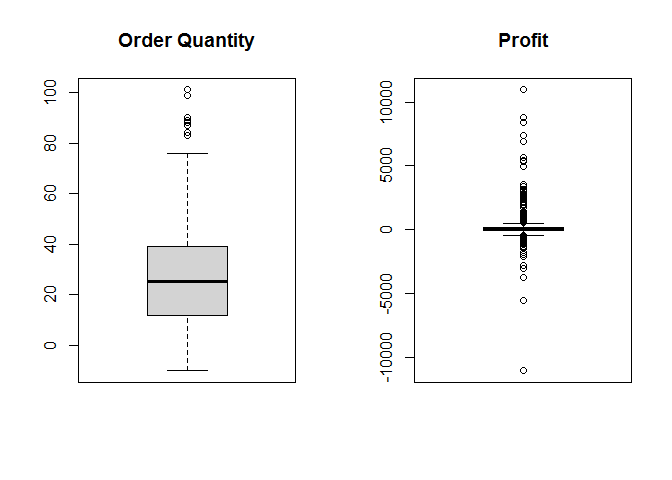boxplot(S1$Sales, main = "Sales")
boxplot(S1$Shipping.Cost, main = "Shipping Cost")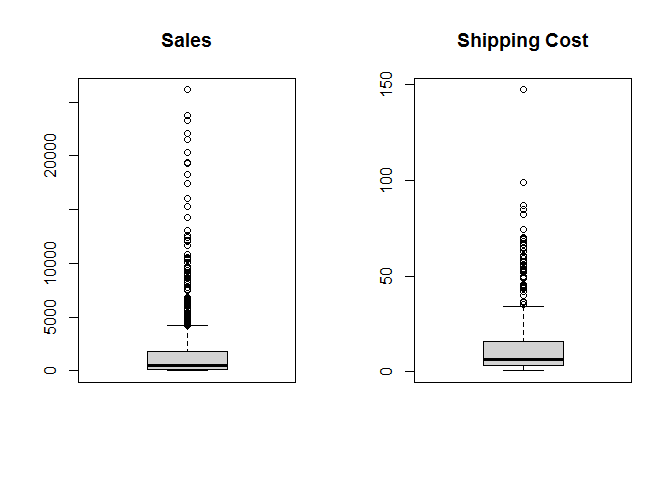boxplot(S1$Unit.Price, main = "Unit Price")
par(mfrow = c(1, 1))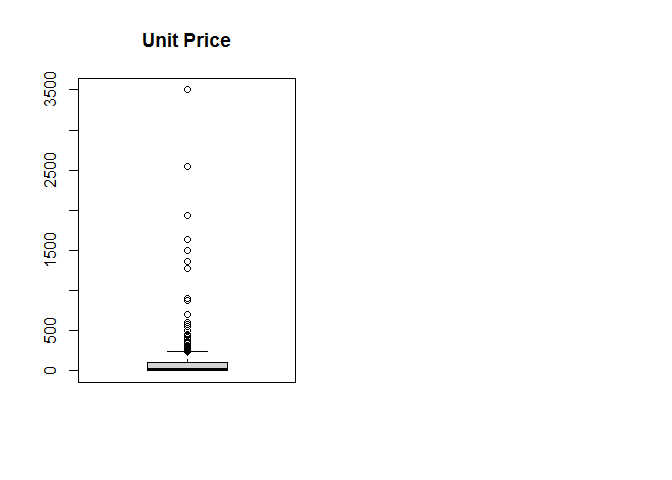All five variables show that there exist outliers.

1. Use any method to identify any outlier/wrong value in Order Priority column. Explain your method.
barplot(summary(S1$Order.Priority))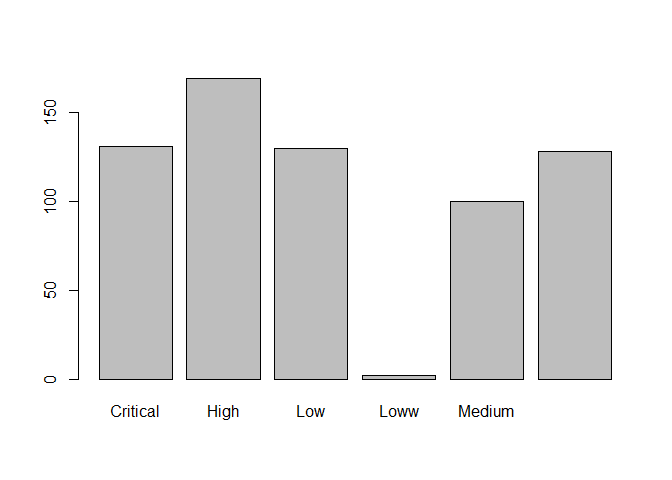I drew a bar plot of values in Order Priority column to see whether there are values that occur very few times compared to other values. It appears that there is a wrong value in Order Priority column - for some observations Order Priority is spelled as “Loww”" instead of “Low”. 1. Remove the rows which contain outliers to get data table (S2). Draw new boxplot(s) for all such variable(s). First, we need to get the indices of all the rows that contain outliers according to the boxplots and then remove those rows from the dataset. ind <- which(S1$Order.Quantity %in% boxplot.stats(S1$Order.Quantity)$out |
S1$Sales %in% boxplot.stats(S1$Sales)$out | S1$Profit %in% boxplot.stats(S1$Profit)$out |
S1$Unit.Price %in% boxplot.stats(S1$Unit.Price)$out | S1$Shipping.Cost %in% boxplot.stats(S1$Shipping.Cost)$out)

S2 <- S1[-ind, ]

We also need to change the Order Priority column values “Loww” to “Low” and then remove the unused levels (“Loww”) with function droplevels:

S2$Order.Priority[S2$Order.Priority == "Loww"] <- "Low"
S2$Order.Priority <- droplevels(S2$Order.Priority)

Finally, we can draw the new boxplots:

par(mfrow = c(1, 2))
boxplot(S2$Order.Quantity, main = "Order Quantity") boxplot(S2$Profit, main = "Profit")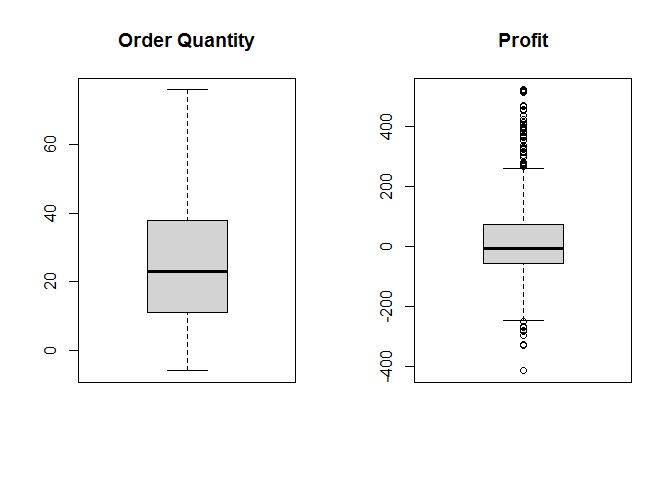boxplot(S2$Sales, main = "Sales") boxplot(S2$Shipping.Cost, main = "Shipping Cost")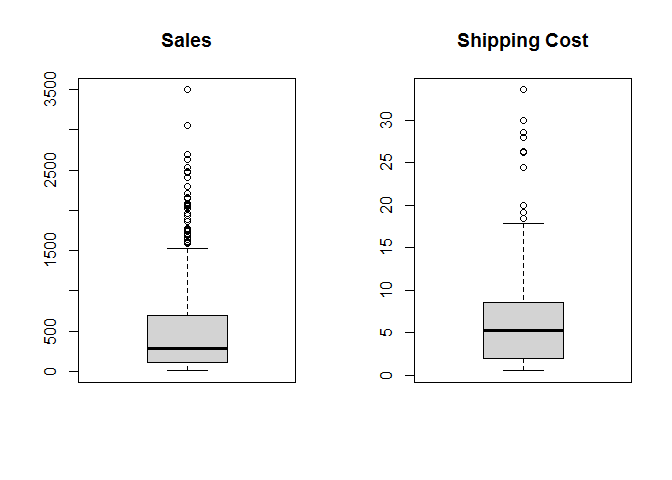boxplot(S2$Unit.Price, main = "Unit Price") par(mfrow = c(1, 1))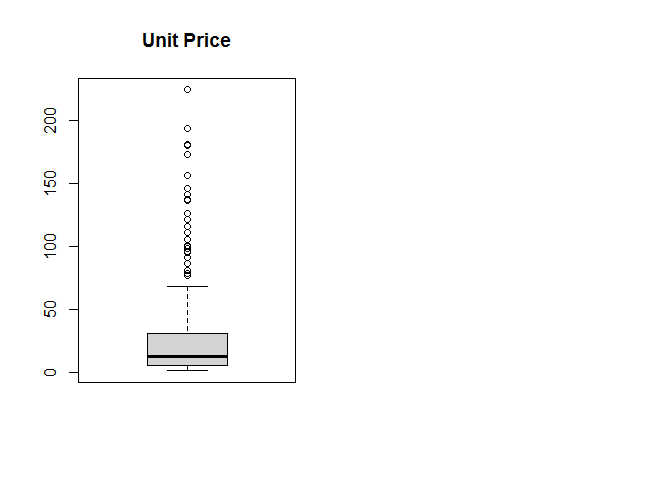1. Standardize all the numeric variables, using (i) z-scores, (ii) min-max normalization, respectively. 1. z-scores S1$Order.Quantity <- scale(S1$Order.Quantity, center = TRUE, scale = TRUE) S1$Sales <- scale(S1$Sales, center = TRUE, scale = TRUE) S1$Profit <- scale(S1$Profit, center = TRUE, scale = TRUE) S1$Unit.Price <- scale(S1$Unit.Price, center = TRUE, scale = TRUE) S1$Shipping.Cost <- scale(S1$Shipping.Cost, center = TRUE, scale = TRUE) 1. min-max normalization S1$Order.Quantity <- (S1$Order.Quantity - min(S1$Order.Quantity)) /
(max(S1$Order.Quantity) - min(S1$Order.Quantity))
S1$Sales <- (S1$Sales - min(S1$Sales)) / (max(S1$Sales) - min(S1$Sales)) S1$Profit <- (S1$Profit - min(S1$Profit)) /
(max(S1$Profit) - min(S1$Profit))
S1$Unit.Price <- (S1$Unit.Price - min(S1$Unit.Price)) / (max(S1$Unit.Price) - min(S1$Unit.Price)) S1$Shipping.Cost <- (S1$Shipping.Cost - min(S1$Shipping.Cost)) /
(max(S1$Shipping.Cost) - min(S1$Shipping.Cost))
1. Use IQR method to identify outliers for numeric variables.
# vector containing the names of numeric variables
vars <- c("Order.Quantity", "Sales", "Profit", "Unit.Price", "Shipping.Cost")
# Create a variable to store the row id's of outliers
outliers <- c()
# Loop through the list of numeric variables
for(i in vars){
# Get the Min/Max values
max <- quantile(S1[, i], 0.75) + (IQR(S1[, i]) * 1.5)
min <- quantile(S1[, i], 0.25) - (IQR(S1[, i]) * 1.5)
# Get the row id's of outliers
id <- which(S1[, i] < min | S1[, i] > max)
# Append the outliers list
outliers <- c(outliers, id)
}
# Rows, that contain outliers
outliers <- sort(unique(outliers))
outliers
##      1   2   4   9  16  18  19  28  36  38  40  44  48  57  60  69  71  74
##    77  80  83  84  85  87  89  90  94 100 104 107 108 109 110 112 113 115
##   116 119 127 130 132 133 135 138 141 142 153 154 155 157 161 162 166 172
##   174 187 198 199 208 209 211 213 214 217 231 235 242 243 249 258 260 265
##   266 267 268 270 271 277 278 280 286 295 297 299 312 317 325 328 335 337
##   340 343 345 346 347 348 350 351 357 361 365 369 371 373 374 381 384 391
##  395 397 398 414 416 419 422 423 426 428 429 430 431 435 442 446 447 448
##  455 456 460 464 465 476 477 485 486 493 499 500 502 505 507 508 515 519
##  521 533 534 537 538 546 547 550 560 566 567 568 574 575 576 578 581 582
##  587 591 592 593 598 599 600 605 607 613 614 628 631 634 642 651 653 654
##  656 658 659

Use the data set S2 for the following tasks.

## 3. Data Exploration II

1. For “Order Priority” and “Product category” two categorical variables, construct a distribution table by R function table(). Make comments on the results you get.
table(S2$Order.Priority, S2$Product.Category)
##
##                 Furniture Office Supplies Technology
##   Critical             18              63         17
##   High                  8              83         25
##   Low                  17              54         27
##   Medium                7              47         18
##   Not Specified        14              59         20

It appears that most often, Office Supplies have High Order Priority while Furniture have either Critical Order Priority or Low Order Priority (or it is Not Specified at all). Technology products seem to have similar values for each Order Priority category.

1. Examine the distribution of all numeric variables using histograms. Make comments on the results you obtain.
hist(S2$Order.Quantity, xlab = "Order Quantity", main = "Order Quantity")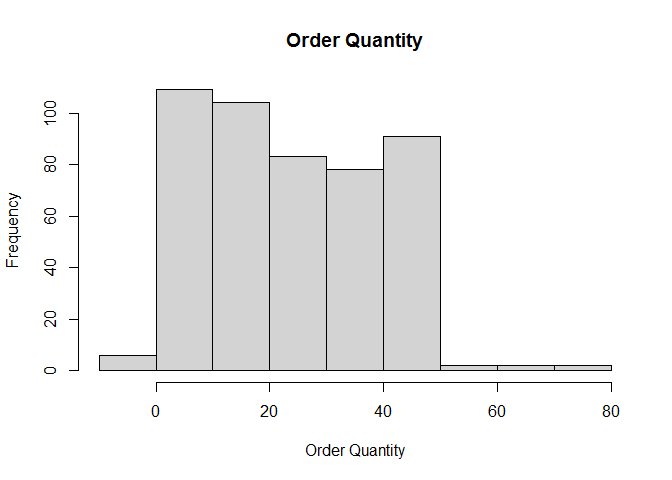Variable Order Quantity seems to be uniformly distributed between 0 and 50 with some outliers. hist(S2$Profit, xlab = "Profit", main = "Profit")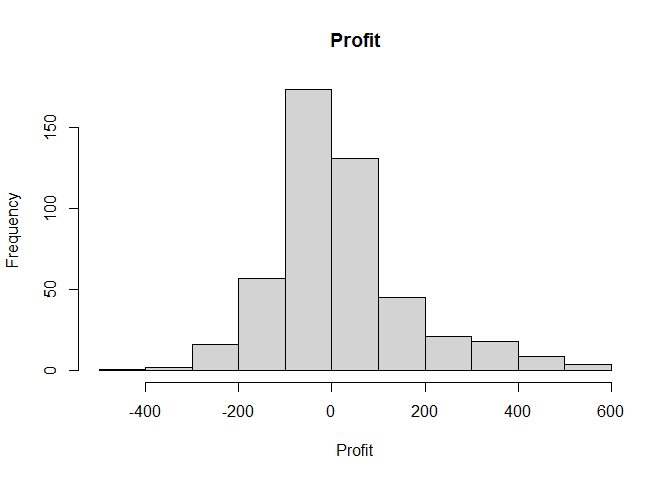Variable Profit appears to be normally distributed because the histogram is symmetric and bell_shaped.

par(mfrow = c(1, 3))
hist(S2$Sales, xlab = "Sales", main = "Sales") hist(S2$Shipping.Cost, xlab = "Shipping Cost", main = "Shipping Cost")
hist(S2$Unit.Price, xlab = "Unit Price", main = "Unit Price")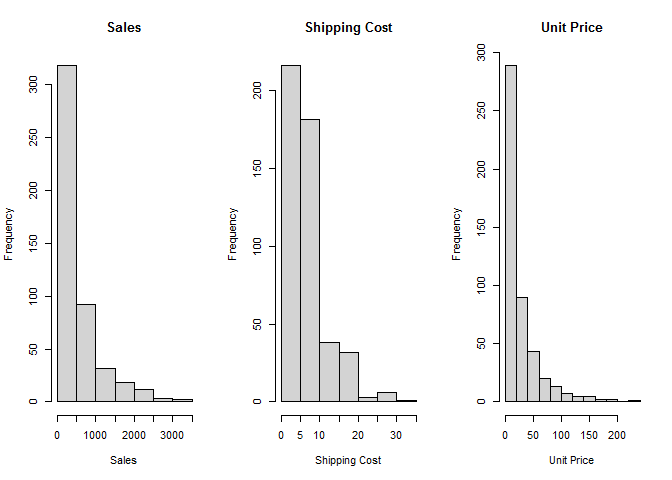par(mfrow = c(1, 1)) Variables Sales, Unit Price and Shipping Cost appear to be exponentially distributed since the histograms are skewed to the right. 1. List the variables in your report which are obviously not normally distributed (in alphabetic order). Implement the transformation to induce approximate normality by square root, inverse square root or nature log. Provide before/after skewness values and quantile-quantile plot for all such variables. Make comments. Variables Sales, Shipping Cost and Unit Price are obviously not normally distributed. # load library for skewness() function library(e1071) Sales before transformation: skewness(S2$Sales)
##  1.993632
qqnorm(S2$Sales) qqline(S2$Sales)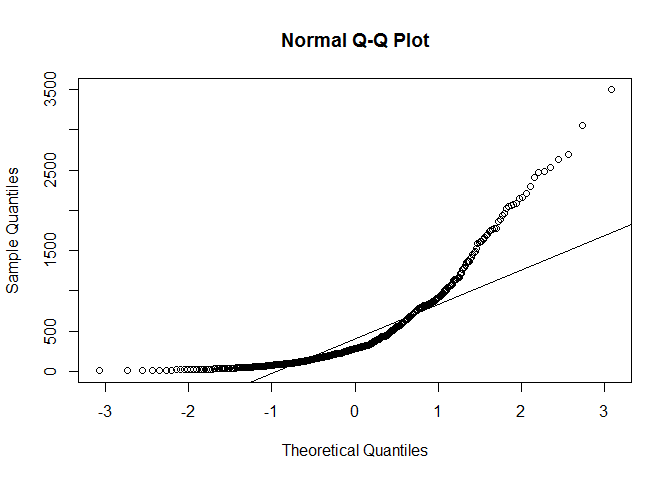Sales after transformation by nature log:

skewness(log(S2$Sales)) ##  -0.3179572 qqnorm(log(S2$Sales))
qqline(log(S2$Sales))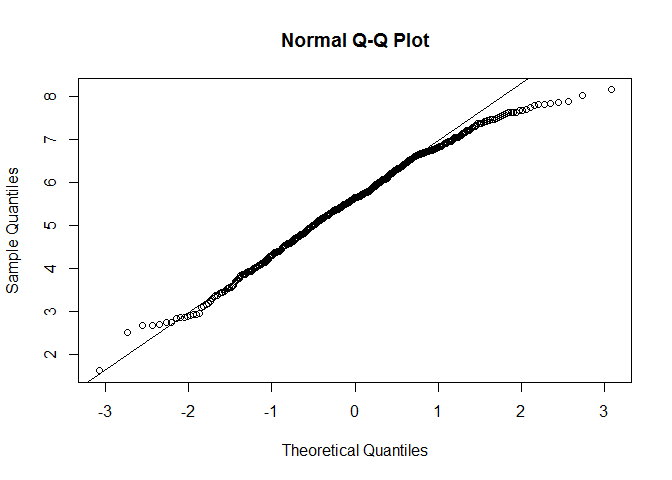Shipping Cost before transformation: skewness(S2$Shipping.Cost)
##  1.641629
qqnorm(S2$Shipping.Cost) qqline(S2$Shipping.Cost)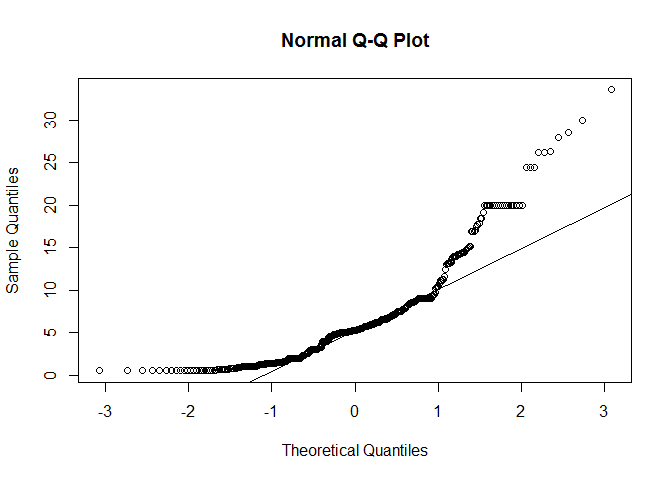Shipping Cost after transformation by nature log:

skewness(log(S2$Shipping.Cost)) ##  -0.4740058 qqnorm(log(S2$Shipping.Cost))
qqline(log(S2$Shipping.Cost))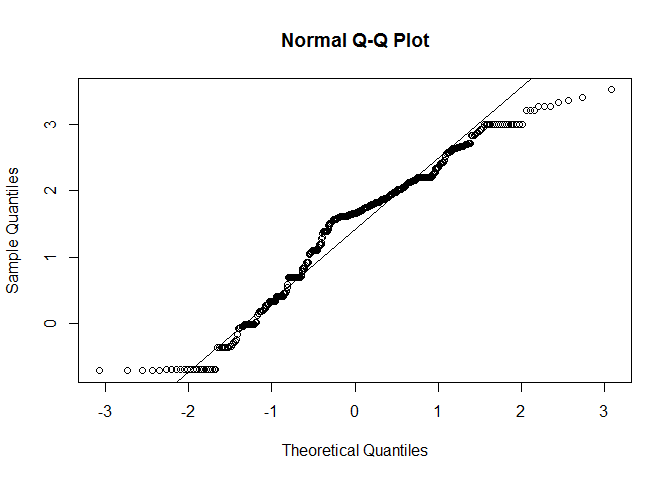Unit Price before transformation: skewness(S2$Unit.Price)
##  2.538852
qqnorm(S2$Unit.Price) qqline(S2$Unit.Price)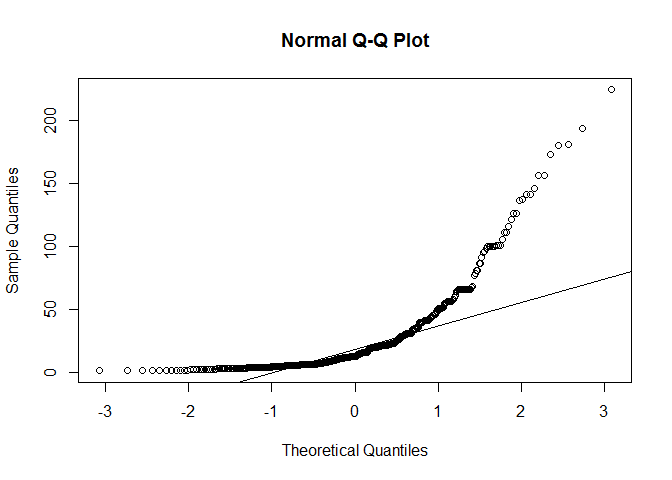Unit Price after transformation by nature log:

skewness(log(S2$Unit.Price)) ##  0.1977216 qqnorm(log(S2$Unit.Price))
qqline(log(S2$Unit.Price))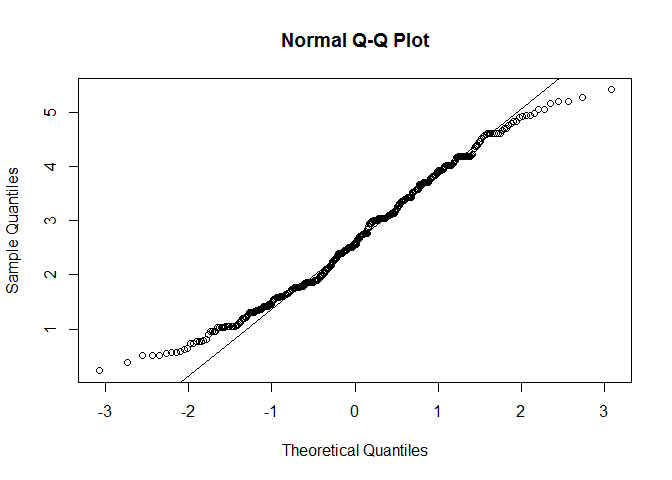Transforming these variables by nature log made them approximately normally distributed. This can be seen from the quantile-quantile plots and decreased skewness value after transformation. ## 4. Creating New Variables 1. Derive one flag variable Profit.flag to indicate whether a product is profitable or not. Construct a distribution table and make your comments. S2$Profit.flag <- S2$Profit > 0 table(S2$Profit.flag)
##
## FALSE  TRUE
##   249   228

There are slightly more products that are not profitable, however the difference is not very large.

1. Derive a new numeric variable, T.cost, to estimate total payment by a customer (unit price * order quantity + shipping cost). Draw a histogram for new variable and comment on that.
S2$T.cost <- S2$Unit.Price * S2$Order.Quantity + S2$Shipping.Cost
hist(S2$T.cost, main = "Histogram of T.cost", xlab = "T.cost")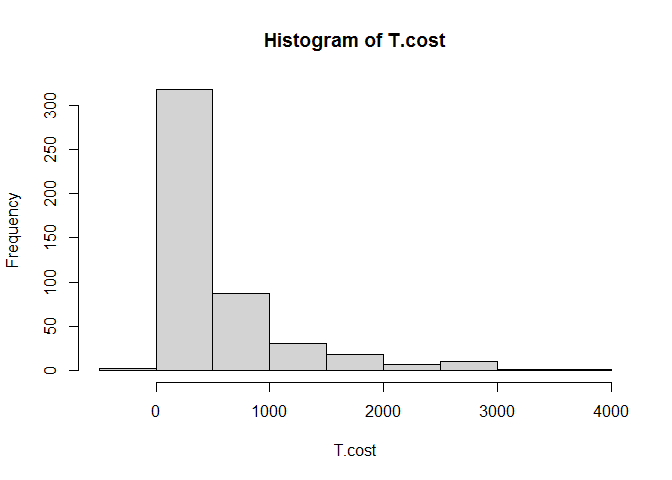Most of the values of T.cost are between 0 and 500, however there are values ranging up to 4000. Also, there seems to be some negative values as well. ## 5. Relationships between Variables 1. Find correlation between shipping cost and order quantity. Draw a scatter plot between them. Make comments on the plot. cor(S2$Shipping.Cost, S2$Order.Quantity) ##  -0.1265484 plot(S2$Order.Quantity, S2$Shipping.Cost, xlab = "Order quantity", ylab = "Shipping cost")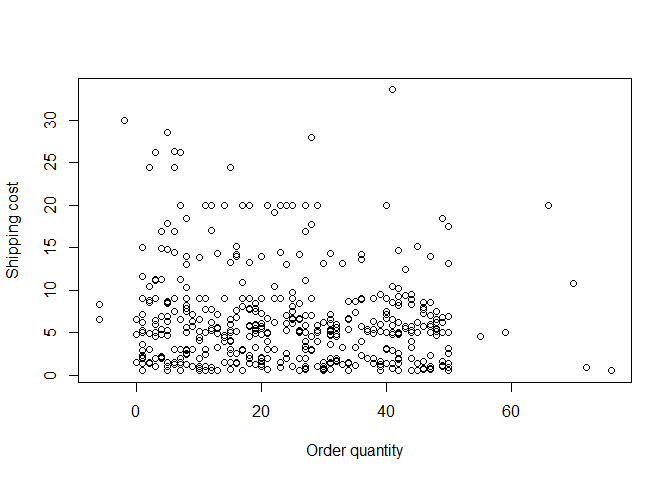There seems to be no relationship between shipping cost and order quantity. 1. Draw a scatter plot between unit price and profit. Make comment on the plot. plot(S2$Unit.Price, S2\$Profit,
xlab = "Unit price", ylab = "Profit")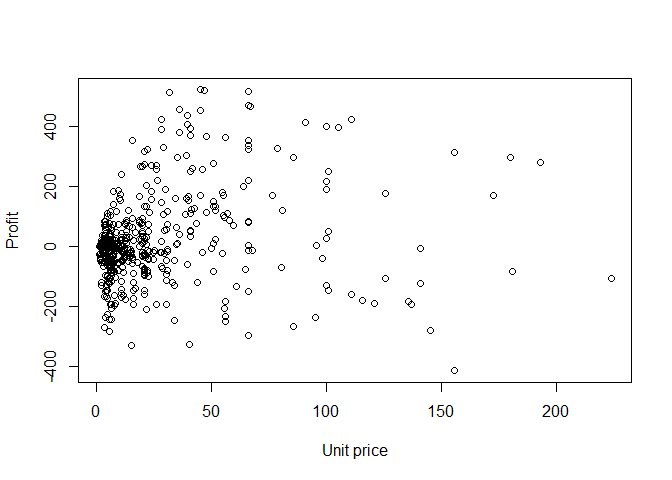There are many points around 0 for both variables, however there seems to be no relationship between unit price and profit.